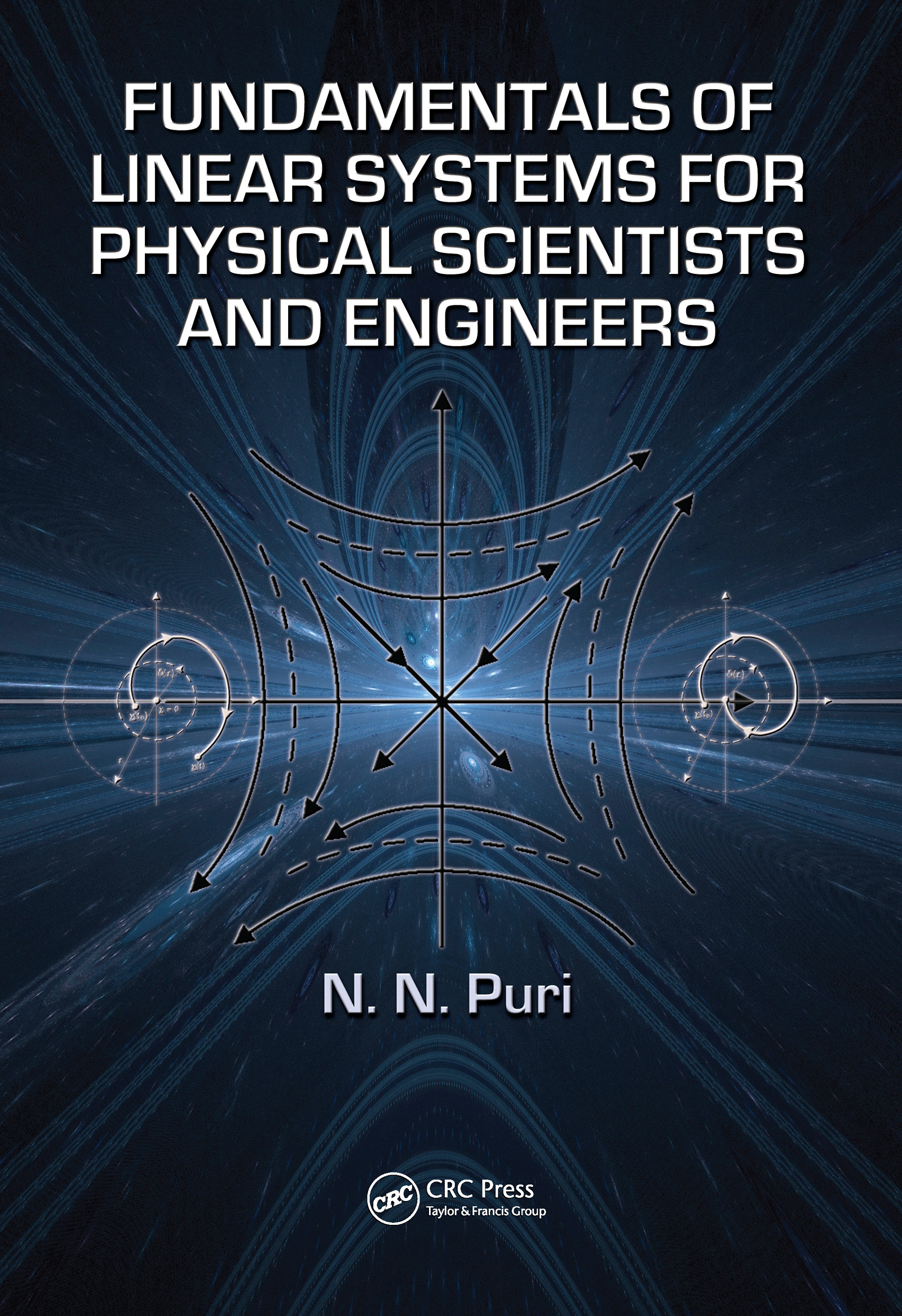# Fundamentals of Linear Systems for Physical Scientists and Engineers

## 1st Edition

CRC Press

899 pages | 16 Color Illus. | 202 B/W Illus.

##### Purchasing Options:\$ = USD
Hardback: 9781439811573
pub: 2009-10-01
Currently out of stock
\$140.00
x
eBook (VitalSource) : 9780429186950
pub: 2009-10-01
from \$35.50

FREE Standard Shipping!

### Description

Thanks to the advent of inexpensive computing, it is possible to analyze, compute, and develop results that were unthinkable in the '60s. Control systems, telecommunications, robotics, speech, vision, and digital signal processing are but a few examples of computing applications. While there are many excellent resources available that focus on one or two topics, few books cover most of the mathematical techniques required for a broader range of applications. Fundamentals of Linear Systems for Physical Scientists and Engineers is such a resource.

The book draws from diverse areas of engineering and the physical sciences to cover the fundamentals of linear systems. Assuming no prior knowledge of complex mathematics on the part of the reader, the author uses his nearly 50 years of teaching experience to address all of the necessary mathematical techniques. Original proofs, hundreds of examples, and proven theorems illustrate and clarify the material. An extensive table provides Lyapunov functions for differential equations and conditions of stability for the equilibrium solutions. In an intuitive, step-by-step manner, the book covers a breadth of highly relevant topics in linear systems theory from the introductory level to a more advanced level. The chapter on stochastic processes makes it invaluable for financial engineering applications.

Reflecting the pressures in engineering education to provide compact yet comprehensive courses of instruction, this book presents essential linear system theoretic concepts from first principles to relatively advanced, yet general, topics. The book’s self-contained nature and the coverage of both linear continuous- and discrete-time systems set it apart from other texts.

System Concept Fundamentals and Linear Vector Spaces

Introduction

System Classifications and Signal Definition

Time Signals and Their Representation

System Input-Output Relations

Signal representation via Linear Vector Spaces

Linear Operators and Matrix Algebra

Introduction

Introduction to Matrix Algebra - Euclidian Vector Space

Systems of Linear Algebraic Equations

Diagonalization - Eigenvalue Decomposition of Matrices

Multiple Eigenvalues and Jordan Canonical Form

Determination of the Co-Efficients of the Characteristic Polynomial of a Matrix

Computation of the Polynomial Function of the Matrix A

S-N Decomposition of a Non-singular Matrix

Computation of An without Eigenvectors

Operator Algebra and Related Concepts (Finite and Infinite Dimensions)

Ordinary Differential and Difference Equations

Introduction

System of Differential and Difference Equations

Matrix Formulation and Solution of n-th Order Differential Equations

Matrix Formulation of the k-th Order Difference Equation

Linear Differential Equations with Variable Coefficients

Summary

Complex Variables for Transform Methods

Introduction

Theory of Complex Variables and Contour Integration

Poisson’s Integral on Unit Circle (or disk)

Positive Real Functions

Integral Transform Methods

Introduction

Fourier Transform Pair Derivation

Another Derivation of Fourier Transform

Derivation of Bilateral Laplace Transform Lb

Another Derivation of the Bilateral Laplace Transform

Single-Sided Laplace Transform (Laplace Transform)

Summary of Transform Definitions

Laplace Transform Properties

Recovery of the Original Time Function from the given Single-Sided Laplace Transform

Solution of Linear Constant Coefficient Differential Equations via The Laplace Transform

Computation of x(t) from X(s) For Causal Processes

Inverse of Bilateral (Two-Sided) Laplace Transform Fb(s)

Transfer Function

Impulse Response

Time Convolution for Linear Time Invariant System

Frequency Convolution in Laplace Domain

Parseval’s Theorem

Generation of Orthogonal Signals in Frequency Domain

The Fourier Transform

Fourier Transform Properties

Fourier Transform Inverse

Hilbert Transform

Application of The Integral Transforms to The Variable Parameter Differential Equations

Generalized Error Function

Digital Systems, Z-Transforms and Applications

Introduction

Discrete Systems and Difference Equations

Realization of a general Discrete System

Z-Transform for the Discrete Systems

Fundamental Properties of Z-Transforms

Evaluation of f (n), given its Single Sided Z-Transform

Solution of Difference Equations using Z-Transforms

Computation Algorithm for the Sum of the Squares of the Discrete Signal Sequence

Bilateral Z-Transform f (n) ↔ Fb(z)

Evaluation of some of the Important Series via Z-Transforms

Reconstruction of a Continous-Time Band-limited Signal from Uniform Samples

State Space Description Of Dynamic Systems

Introduction

State Space Formulation

Selection of The State Variables and Formulation of The State Space Equations

Methods of Deriving State Variable Equations for The Physical System

State Space Concepts

Calculus Of Variations

Introduction

Calculus of Maxima, Minima and stationary points (Extrema of a Function)

Extremal of a Function subject to Multiple Constraints

Extremal of a Definite Integral - Derivation of Euler-Lagrange Equations with variable end points

Extremal of a Definite Integral with Multiple Constraints

Mayer Form

Bolza’s Form

Variational Principles and Optimal Control

Hamilton-Jacobi Formulation of Euler-Lagrange Equations

Pontryagin’s Extremum Principle

Dynamic Programming

Stochastic Processes and Linear Systems Response to Stochastic Inputs

Preliminaries

Continous Random Variable and probability density function (pdf)

Random Walk, Brownian Motion and Wiener Process

Markov Chains, Inequalities and Law of Large Numbers

Stochastic Hilbert Space

Random or Stochastic Processes

Wiener Filters

Optimal Estimation, Control, Filtering and Prediction - Continuous Kalman Filters

### About the Author

N.N. Puri is Professor of Electrical and Computer Engineering at Rutgers University, New Jersey.

### Subject Categories

##### BISAC Subject Codes/Headings:
TEC007000
TECHNOLOGY & ENGINEERING / Electrical
TEC009000
TECHNOLOGY & ENGINEERING / Engineering (General)